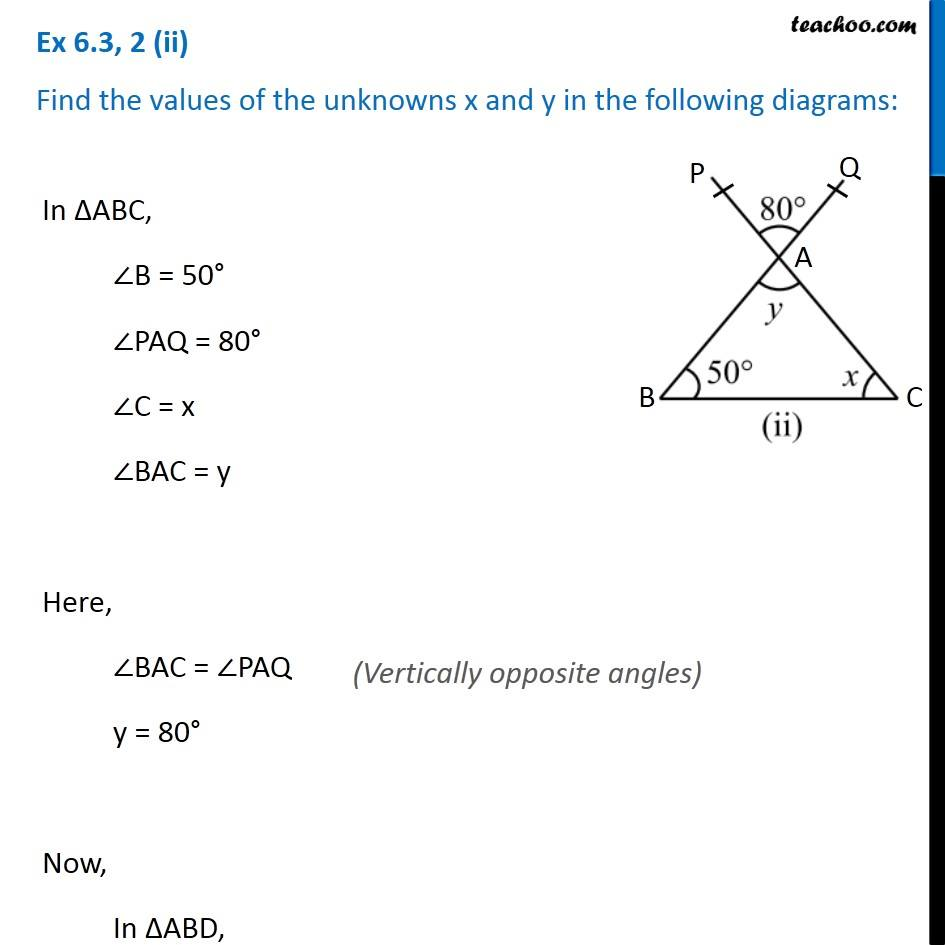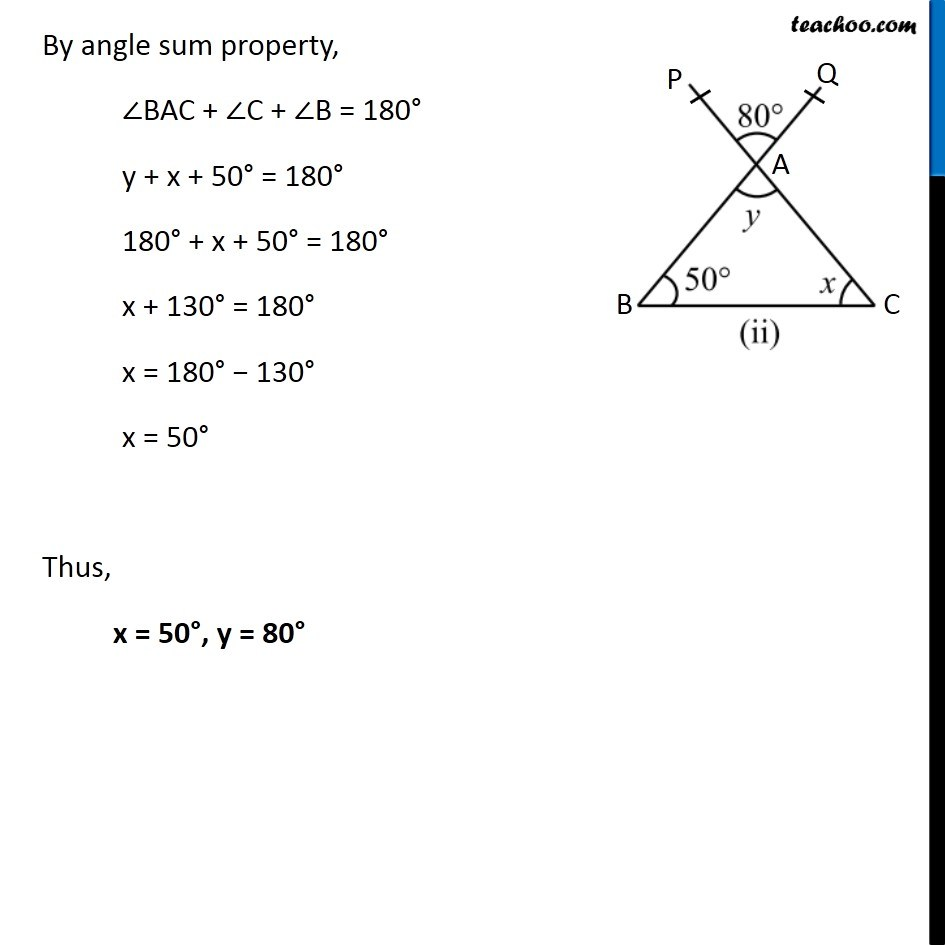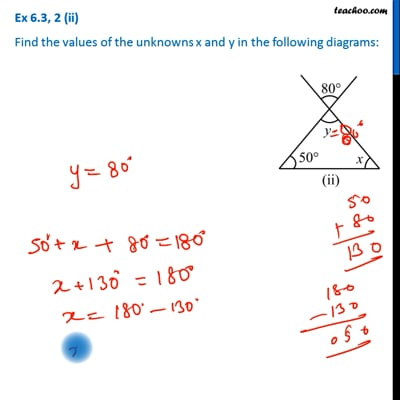Ex 6.3

Chapter 6 Class 7 Triangle and its Properties
Serial order wiseThis video is only available for Teachoo black users

Get live Maths 1-on-1 Classs - Class 6 to 12

### Transcript

Ex 6.3, 2 (ii) Find the values of the unknowns x and y in the following diagrams:In ∆ABC, ∠B = 50° ∠PAQ = 80° ∠C = x ∠BAC = y Here, ∠BAC = ∠PAQ y = 80° Now, In ∆ABD, By angle sum property, ∠BAC + ∠C + ∠B = 180° y + x + 50° = 180° 180° + x + 50° = 180° x + 130° = 180° x = 180° − 130° x = 50° Thus, x = 50°, y = 80°